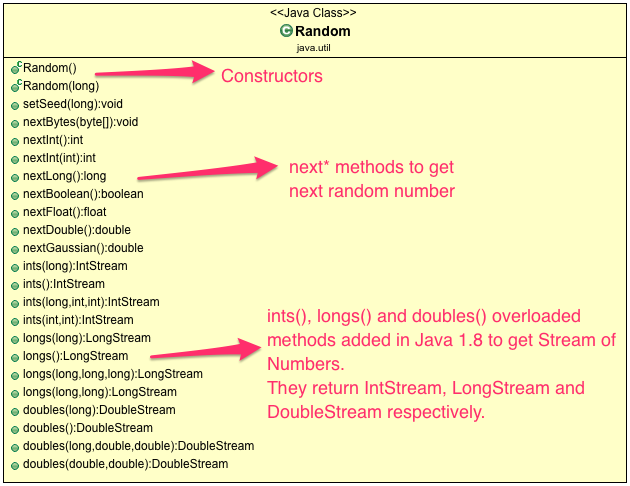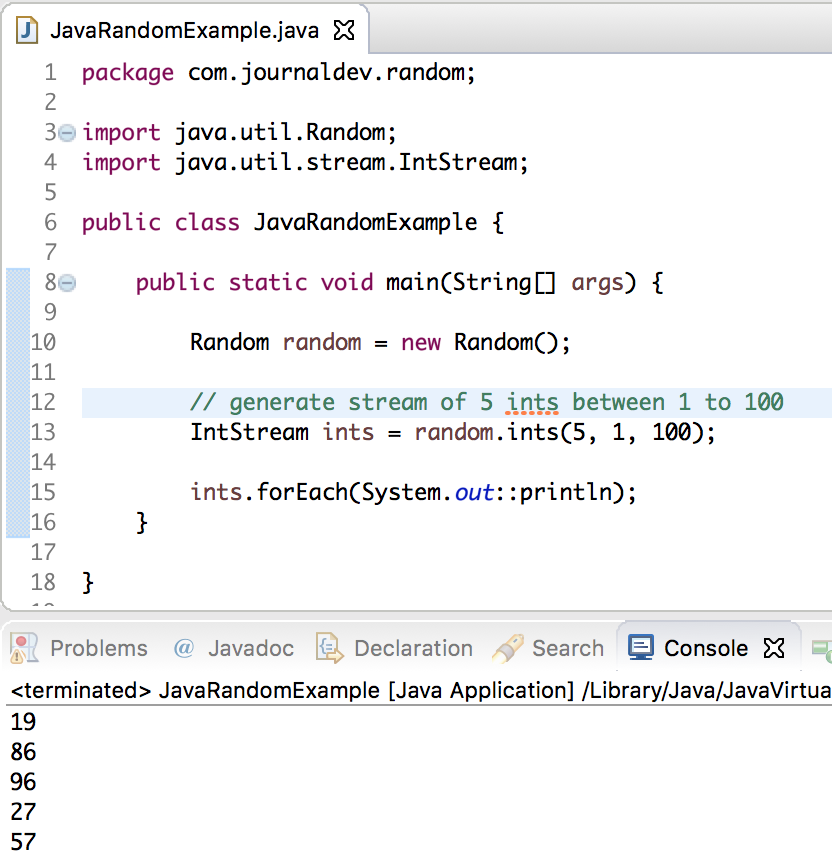# Java Random

Published on August 3, 2022By PankajWhile we believe that this content benefits our community, we have not yet thoroughly reviewed it. If you have any suggestions for improvements, please let us know by clicking the “report an issue“ button at the bottom of the tutorial.

Java Random class is used to generate a series of random numbers.

## Java Random Class

• `Random` class is part of java.util package.
• An instance of java Random class is used to generate random numbers.
• This class provides several methods to generate random numbers of type integer, double, long, float etc.
• Random number generation algorithm works on the seed value. If not provided, seed value is created from system nano time.
• If two Random instances have same seed value, then they will generate same sequence of random numbers.
• Java Random class is thread-safe, however in multithreaded environment it’s advised to use `java.util.concurrent.ThreadLocalRandom` class.
• Random class instances are not suitable for security sensitive applications, better to use `java.security.SecureRandom` in those cases.### Java Random Constructors

Java Random class has two constructors which are given below:

1. `Random()`: creates new random generator
2. `Random(long seed)`: creates new random generator using specified seed

### Java Random Class Methods

Let’s have a look at some of the methods of java Random class.

1. `nextBoolean()`: This method returns next pseudorandom which is a boolean value from random number generator sequence.
2. `nextDouble()`: This method returns next pseudorandom which is double value between 0.0 and 1.0.
3. `nextFloat()`: This method returns next pseudorandom which is float value between 0.0 and 1.0.
4. `nextInt()`: This method returns next int value from random number generator sequence.
5. nextInt(int n): This method return a pseudorandom which is int value between 0 and specified value from random number generator sequence.

### Java Random Example

Let’s have a look at the below java Random example program.

``````package com.journaldev.examples;

import java.util.Random;

/**
* Java Random Number Example Program
*
*/
public class RandomNumberExample {

public static void main(String[] args) {

//initialize random number generator
Random random = new Random();

//generates boolean value
System.out.println(random.nextBoolean());

//generates double value
System.out.println(random.nextDouble());

//generates float value
System.out.println(random.nextFloat());

//generates int value
System.out.println(random.nextInt());

//generates int value within specific limit
System.out.println(random.nextInt(20));

}

}
``````

Output of the above program is:

``````false
0.30986869120562854
0.6210066
-1348425743
18
``````

### Generate Random Number using Seed

There are two ways we can generate random number using seed.

``````Random random = new Random(long seed);

Random random1 = new Random();
random1.setSeed(seed);
``````

The seed is the initial value of the internal state of the pseudorandom number generator which is maintained by method next(int).

``````package com.journaldev.examples;

import java.util.Random;

/**
* Java Random Number Using Seed Example Program
*
* @author pankaj
*
*/

public static void main(String[] args) {

//using constructor
Random random = new Random(100);
System.out.println("Using Constructor");
System.out.println(random.nextInt());

//using method
Random random2 = new Random();
random2.setSeed(200);
System.out.println("Using Method");
System.out.println(random2.nextInt());
}
}
``````

Output of the above program is:

``````Using Constructor
-1193959466
Using Method
-1133938638
``````

What if we pass same seed to two different random number generators? Let’s have a look at the below program and see what happen if we pass same seed to two different random number generators.

``````package com.journaldev.examples;

import java.util.Random;

/**
* Java Random Number Using Same Seed Example Program
*
*/
public class RandomNumberSameSeedExample {

public static void main(String[] args) {

//initialize two random number generators using same seed
Random random1 = new Random(100);
Random random2 = new Random(100);

System.out.println(random1.nextInt());
System.out.println(random2.nextInt());

}

}
``````

Output of the above program is:

``````-1193959466
-1193959466
``````

We can see that it will generate same random number if we pass same seed to two different random number generators.

### Java 8 Random Class Methods

As you can see from above image, there are many new methods added in Java 8 to Random class. These methods can produce a stream of random numbers. Below is a simple program to generate a stream of 5 integers between 1 and 100.

``````package com.journaldev.random;

import java.util.Random;
import java.util.stream.IntStream;

public class JavaRandomExample {

public static void main(String[] args) {

Random random = new Random();

// generate stream of 5 ints between 1 to 100
IntStream ints = random.ints(5, 1, 100);

ints.forEach(System.out::println);
}

}
``````That’s all for a quick roundup on Java Random Class. Reference: API Doc

Thanks for learning with the DigitalOcean Community. Check out our offerings for compute, storage, networking, and managed databases.Pankaj

author

#### Still looking for an answer?

Ask a questionSearch for more help

Click below to sign up and get \$200 of credit to try our products over 60 days!

### Popular Topics##### Hollie's Hub for Good

Working on improving health and education, reducing inequality, and spurring economic growth? We’d like to help.##### Become a contributor

You get paid; we donate to tech nonprofits.

##### Welcome to the developer cloud

DigitalOcean makes it simple to launch in the cloud and scale up as you grow – whether you’re running one virtual machine or ten thousand.© 2023 DigitalOcean, LLC.